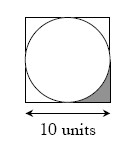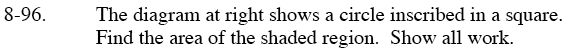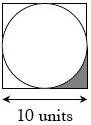Home > GC > Chapter 8 > Lesson 8.3.2 > Problem8-96

8-96.

The diagram below shows a circle inscribed in a square. Find the area of the shaded region. Show all work.
Homework Help ✎Find the area of the circle.

π r2 = π (5)2

Find the area of the square.

Subtract the area of the circle from the area of the square to find the area of all four corners.

Divide the answer for the previous step by 4 to find the area of the shaded region.